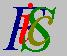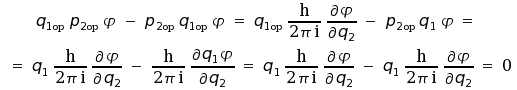Mauro Murzi's pages on Philosophy of Science - Quantum mechanics
Commutative operatorsAlpha radioactivity. Non commutative operators# 5. Heisenberg indeterminacy principle.

The section begins with the distinction between commutative and non commutative operators. The role of this distinction, which is a main feature of quantum mechanics, is illustrated by the analysis of measurement in quantum mechanics. A brief explanation of Ehrenfest theorem on mean values precedes the formulation of Heisenberg indeterminacy principle. The section ends with some physical applications of Heisenberg indeterminacy principle.

## Commutative operators.

Let u and v be two physical quantities, and let uop and vop be the associated quantum operators. Suppose that uop is applied to a complex function φ to construct the function uop φ . It is possible to apply vop to that function and obtain vop uop φ ; this expression indicates the application of operator uop to the function φ , followed by the application of operator vop to the resulting function.

The question arise whether the order of application of quantum operators is essential: vop uop φ is the same as uop vop φ ? If you remember the meaning of uop vop φ , which is not a multiplication of number, but an application of quantum operators to transform a function in another function, you can guess that in general vop uop φ is not the same as uop vop φ . However, for some quantum operators uop and vop , the following relation holds:

(5.1)    vop uop φ = uop vop φ

Two particular quantum operators for which the relation (5.1) is true for every function φ are called commutative operators, and the corresponding physical quantities are called commutative entities. Note that the notion of commutative operators is applicable only to a pair of quantum operators: An operator is commutative with respect to another operators.

An as example of a pair of commutative quantum operators, consider the position q1 and the momentum p2 ; note that they are referred to two different axes. We have:There is an important property which holds for a pair of commutative entities: Two commutative entities have the same set of eigenfunctions.Alpha radioactivity. Non commutative operators